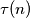### IMO Shortlist 2004 problem N1

Kvaliteta:
Avg: 0,0
Težina:
Avg: 6,0
Let$\tau(n)$ denote the number of positive divisors of the positive integer$n$. Prove that there exist infinitely many positive integers$a$ such that the equation$\tau(an)=n$ does not have a positive integer solution$n$.
Izvor: Međunarodna matematička olimpijada, shortlist 2004